All good with your if and else machine learning system?

It was a joke but this one made me think.

This is a running gag: machine learning is only a series of if and else.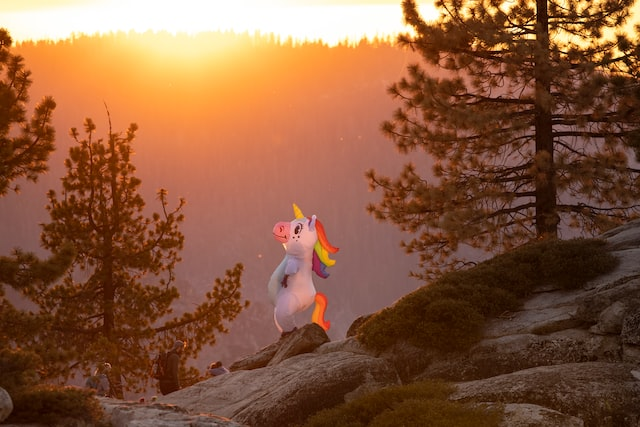Beyond the joke, it is true?

Yes! …and no. As always, it depends.

Quick answer: Machine learning is a bunch of mathematical and statistical operations. Sometimes, the operations you use can be translated into if and else clauses, and sometimes not. But you never write the series of if and else yourself.

## A recap of machine learning

The idea of machine learning is: you have some data, and you apply an algorithm to these to detect a pattern. You put this pattern into a function.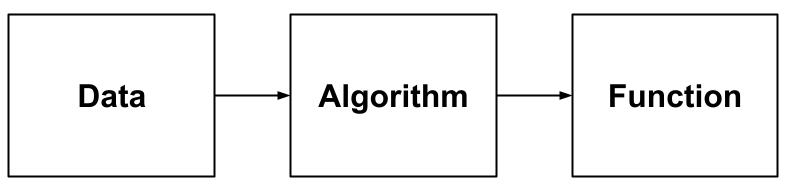Then, you’ll be able to use this function on new data to extract new information.

## A decision tree with a series of if and else

There are different types of machine learning. If you decide to build a decision tree (a famous way to do machine learning) to know the form of a diamond, you’ll get something like that: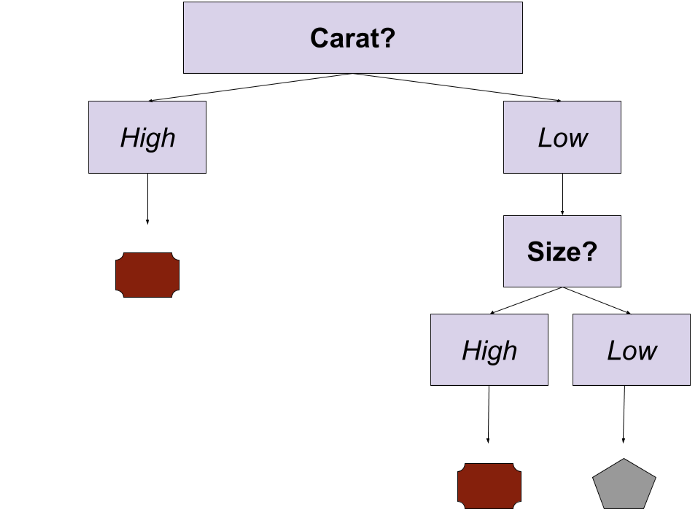If you translate it with code, you’ll get something like that:

if carat (the weight of a diamond) is high:
return red plate
else:
if size is high:
return red plate
else:
return grey pentagon


Then, yes, you can see that here, you have a series of if and else.

And decision trees are used a lot in machine learning. Most of the time, you don’t use decision trees directly but forests of decision trees in the Random Forest algorithm or a series of decision trees in the Gradient Boosted Trees algorithm.

## But, many algorithms in machine learning are just the generation of plain mathematical formulas

Let’s take another famous way to do machine learning: a neural network. What you’ll get at the end is more something like that:

a*10+b*15+c*16+20…


Then, the process doesn’t try to find a series of if and else, but a mathematical formula.

I would like to finish with a last example: recommender systems. There are many ways to build a recommendation system. One which is well known is matrix factorization.

Matrix factorization, what?

I won’t explain deeply what it is about, but as a sum up, it’s a manipulation of matrices. It comes from linear algebra. Here is a definition: Matrix decomposition.

The result is something like that:

Vector A * Vector B


As a result, yes, you have types of machine learning that will generate a series of if and else. But, you have also plenty of algorithms that try to find the variables of an equation or vectors.

## You never write the series of if and else yourself

Let’s go back to the decision tree. As you’ve seen previously, the result could be translated as a series of if and else.

But, you don’t write directly this code. You generate it using… mathematical operations. Yes, again!

As an example, you can get the result of a decision tree using an optimisation algorithm with the Shannon Entropy formula: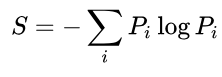Let’s suppose you want to guess the form (pentagon or plate) of a diamond according to its attributes. You have three diamonds:

carat size form
high small plate
low high plate
low small pentagon

The process is the following:

1. The data is split randomly: a random if statement is created like if carat is high
2. The process checks if it helps to generate a more accurate view of the data: by doing this if, are the data separated correctly? Do we have pentagons mostly from one side and plates from another?

To be able to know if the data are separated correctly, the Shannon entropy formula is used

• if yes, the process keeps the if carat is high
• if not, it generates another one

Then, by keeping the if you get something like that: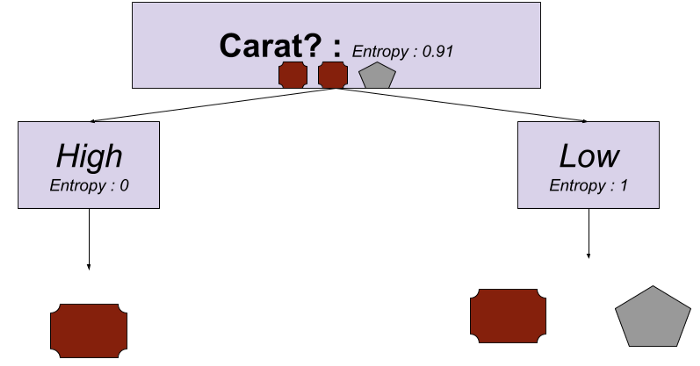The translation with a code is:

if carat (the weight of a diamond) is high:
return red plate
else:
#The process doesn't know yet how to handle that


Note that you have a branch (below low) with a plate and a pentagon. It corresponds to the else where the process doesn’t know what to put yet.

1. So, the data below low is split randomly: another if is created
2. The process checks if it helps to generate a more accurate view of the data
• if yes, the process keeps the new if
• if not, it generates another one

By keeping the new if, you get another branch: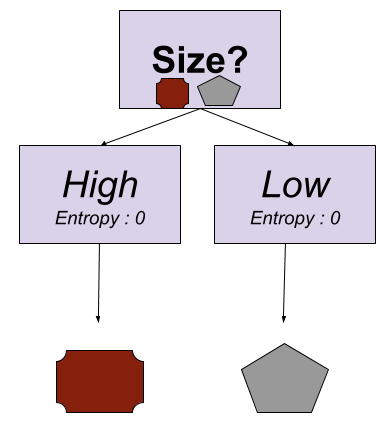The translation with a code is:

if size is high:
return red plate
else:
return grey pentagon


At the end, you get a final tree decision:with a final code:

if carat (the weight of a diamond) is high:
return red plate
else:
if size is high:
return red plate
else:
return grey pentagon


Of course, in real life, data are more complicated and the process must iterate a lot until getting the perfect tree. The process used is an optimisation algorithm. This is the part called learning in machine learning.

Mathematical optimization […] is the selection of a best element, with regard to some criterion, from some set of available alternatives (definition from Wikipedia)

If you want to know how the Shannon entropy works with mathematical formulas, you’ve got this article: Classification in machine learning - Example of Decision Tree with Shannon Entropy

Then as a result, yes, you can have machine learning algorithms that will build a series of if and else. But to generate it, you’ll use mathematical operations.

Note that for other algorithms such as the matrix factorisation or neural networks, you don’t use a process with the Shannon entropy formula, but other optimisation algorithms that don’t generate a series of if and else but, as previously seen, vectors or formulas.

## So why do we sometimes say that machine learning is a bunch of if and else statements?

To my opinion, because of expert systems. They are the ancestors of machine learning in artificial intelligence.

Artificial intelligence is a way to simulate human cognitive abilities. In the history of artificial intelligence, people thought that they would be able to target that with expert systems. These are big series of hardcoded rules and then… of if and else.

## Conclusion

To conclude, most of the time, machine learning is not a series of if and else. It’s just mathematics and for some techniques, they are very old. I’m thinking of linear regressions or Bayesian probabilities. These were used long before the existence of computers.

Photo of the unicorn by Stephen Leonardi on Unsplash